Ch 2. Fluid Statics Multimedia Engineering Fluids PressureVariation PressureMeasurement HydrostaticForce(Plane) HydrostaticForce(Curved) Buoyancy
 Chapter 1. Basics 2. Fluid Statics 3. Kinematics 4. Laws (Integral) 5. Laws (Diff.) 6. Modeling/Similitude 7. Inviscid 8. Viscous 9. External Flow 10. Open-Channel Appendix Basic Math Units Basic Equations Water/Air Tables Sections Search eBooks Dynamics Fluids Math Mechanics Statics Thermodynamics Author(s): Chean Chin Ngo Kurt Gramoll ©Kurt GramollFLUID MECHANICS - THEORY The concept of absolute and gage pressure is discussed in this section. Several common pressure measurement devices and techniques are also introduced.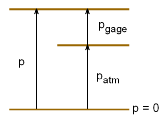Gage Pressure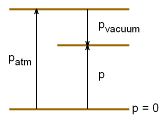Vacuum Pressure Absolute and Gage Pressure When pressure is measured relative to absolute zero pressure, it is called absolute pressure. On the other hand, pressure measuring devices often use gage pressure, which is measured relative to atmospheric pressure. Standard atmospheric pressure at sea level is 101.3 kPa or 14.7 psi. Gage pressure is referred to as vacuum or suction pressure when it is negative. Gage Pressure:        pgage = pabsolute - patm Vacuum Pressure:    pvacuum = patm - pabsolute For example, when the driver's manual for your car suggests that you keep your tires at a pressure of 30 psi, it is refering to the gage pressure. This is equivalent to 44.7 psi absolute pressure. Barometer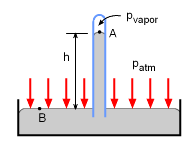Mercury Barometer Atmospheric pressure can be measured through a mercury barometer. A simple barometer consists of an inverted glass tube filled with mercury with its open end submerged in a mercury container. According to the hydrostatic pressure distribution derived in the last section, the atmospheric pressure is given by      patm = ρgh + pvapor The vapor pressure in the glass tube is negligibly small, hence the atmospheric pressure is simply given by the height of the mercury column:      patm = ρgh For example, a column height of 760 mm Hg corresponds to the standard atmospheric pressure of 101.3 kPa (14.7 psi) at sea level. If water is substituted for mercury, then a column height of 10.3 m H2O is needed for atmospheric pressure. Piezometer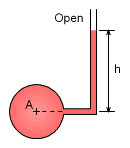Piezometer A piezometer is the simplest form of a pressure measuring device. It has a vertical tube connected to the container in which the pressure is needed. The pressure head of the fluid column indicates the pressure of the container:      pA = ρgh where pA is the gage pressure at point A within the container. The disadvantages of piezometers are: (1) Cannot measure vacuum pressure since air would be sucked into the container through the tube. (2) The measured pressure should be reasonably low, otherwise a very long vertical tube is needed. Manometer Another pressure measuring device is the manometer. It consists of a U-tube with one end connected to the container with an unknown pressure and the other end open to the known atmospheric pressure. The fluid in the U-tube manometer (gage fluid) can be different from the fluid in the container. The procedure for determining the pressure inside the container is: (1) Start from one end, and work from one fluid level to another, up to the open end of the manometer. (2) Remember that pressure increases linearly with depth for a fluid at rest.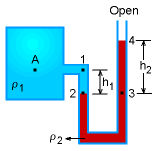U-Tube Manometer Consider the U-tube manometer shown. The pressure at point A inside the tank is calculated as:      pA + ρ1gh1 - ρ2gh2 = 0 which gives:         pA = ρ2gh2 - ρ1gh1 Once again, gage pressure is used in the above equation (i.e., theatmospheric pressure at the open end is zero gage). If the fluid in the tank is a gas, then the pressure between point 1 and 2 is negligible, hence      pA = ρ2gh2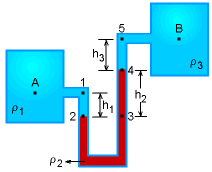Differential U-Tube Manometer The U-tube manometer also can be used to determine the pressure difference between two systems. This type of manometer is called a differential U-tube manometer. Consider the differential manometer connected between tanks A and B, as shown in the figure. The pressure will be determined by moving from point A to point B:      pA + ρ1gh1 - ρ2gh2 - ρ3gh3 = pB The pressure difference is given by      pA - pB = ρ2gh2 + ρ3gh3 - ρ1gh1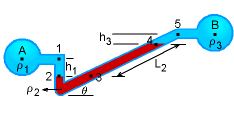Inclined-Tube Manometer Another type of manometer is the inclined-tube manometer which is used to measure small pressure differences between two systems (say for gases). The advantage of the inclined manometer is that the differential reading scales along the tube can be made large compared to a vertical manometer for a given pressure difference, hence improving the accuracy in reading the scale. The pressure difference between point A and B is given by      pA + ρ1gh1 - ρ2gL2sinθ - ρ3gh3 = pB For cases where the columns h1 and h3 are gas, the weights can be neglected, simplifying the equation      pA - pB = ρ2gL2 sinθ

Practice Homework and Test problems now available in the 'Eng Fluids' mobile app
Includes over 250 problems with complete detailed solutions.
Available now at the Google Play Store and Apple App Store.